# Test Heteroskedasticity Glejser Using SPSS

Test Heteroskedasticity Glejser Using SPSS | Heteroskedasticity useful to examine whether there is a difference in the residual variance of the observation period to another period of observation. A Good regression model is not the case heteroscedasticity problem. Many statistical methods that can be used to determine whether a model is free from the problem of heteroscedasticity or not, such as White Test, Test Park, and Test Glejser.

SPSS Test will introduce one of heteroscedasticity test that can be applied in SPSS, namely Test Glejser. Glejser test conducted by regressing absolud residual value of the independent variable with regression equation is: Ut = A + B Xt + vi

Making process in Heteroskedasticity Test with Test Glejser
1. If the value Sig. > 0.05, then there is no problem of heteroscedasticity
2. If the value Sig. <0.05, then there is a problem of heteroscedasticity

Example Test Case in Heteroskedasticity

A company manager wants to know whether the regression model Heteroskedasticity problem occurs or not. The title of the proposed research is "Influence of Competence and Motivation on Employee Performance". For the manager of the collected data competence, motivation and performance of employees from 40 samples. The research data as shown below.Step By Step to Test Glejser Using SPSS

1. Turn on the SPSS program and select the Variable View, next, in the Name write Competency, Motivation, Performance. Ignore the other options.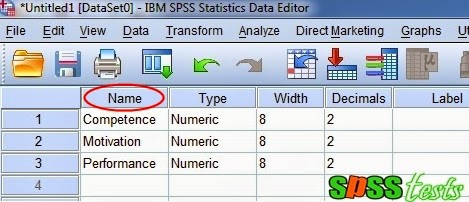2. The next step, click the Data View and enter research data in accordance with the variable Competency, Motivation, Performance.3. Next, from the SPSS menu select Analyze, and then click Regression and then click Linear…4. Then a new dialog box will appear with the name of Linear Regression, then enter the variable competence and motivation to box Independent (s): then enter into the box Dependent variable performance:, and then click Save5. The dialog box appears with the name of Linear Regression: Save, to part Residuals provide a check on the unstandardized, ignore the others, then click the Continue button and then click OK, ignore the output that appears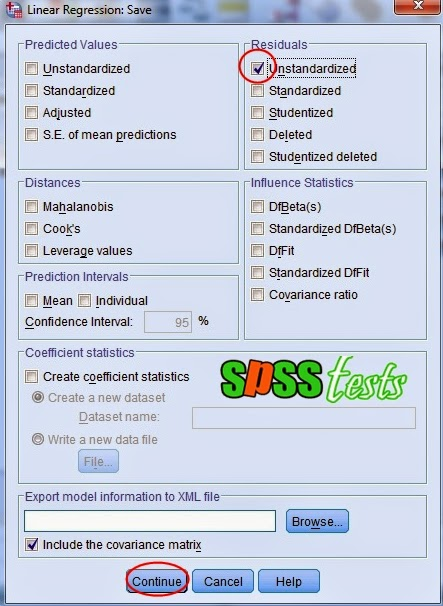6. The next step of the SPSS menu select Transform, and then click the Compute Variable...7. The dialog box appears with the Compute Variable name, the Target Variable box: write AbsUt, then the Numeric Expression box write ABS (RES_1), and then click Ok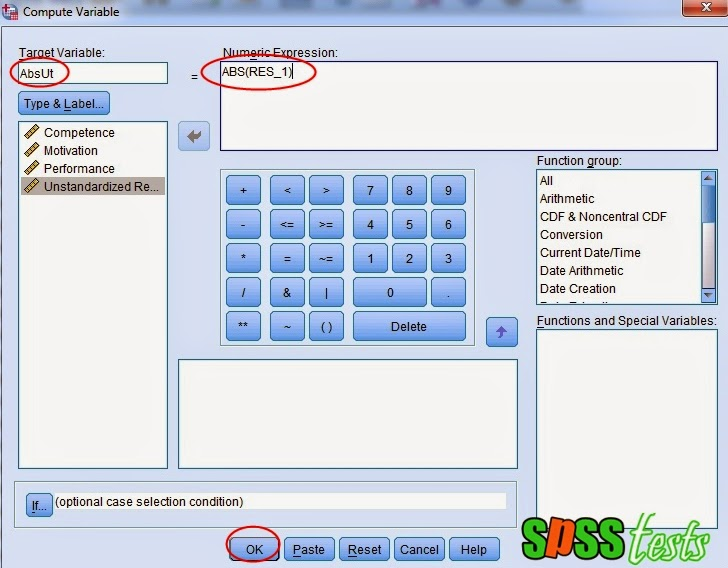8. Next, from the SPSS menu select Analyze, and then click Regression and then click Linear...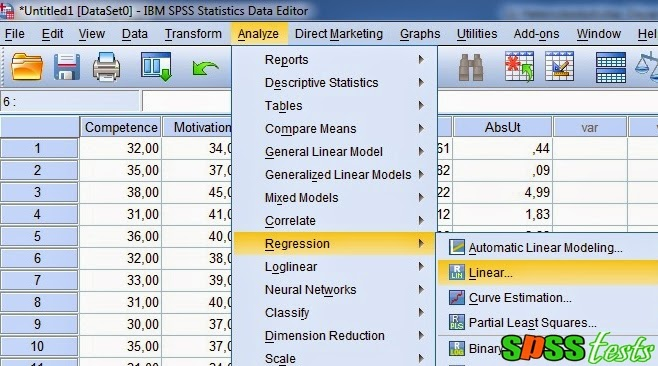9. Then a new dialog box will appear with the name of Linear Regression, then enter the variable competence and motivation to box Independent (s), then remove the first variable and enter the variable performance AbsUt to the Dependent box, then click Save10. Dialog box appears with the name of Linear Regression: Save, to part Residuals unchecks unstandardized section, and then clicks the Continue button11. The last step clicks on Ok to terminate the command, after which it will appear SPSS output, as follows: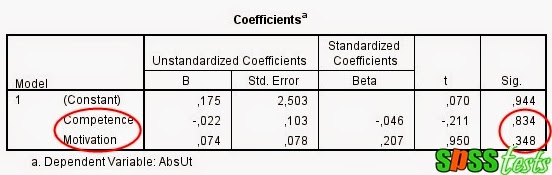Interpretation of Test Results Output Glejser

Based on Output Coefficients the obtained value of Sig. Competence variable of 0.834, and the Sig. Motivation variable of 0.348, meaning that the value of the variable sig Competence and Motivation > 0.05, it can be concluded that there is no heteroscedasticity problem.

Next: Correlation Pearson Product Moment Using SPSS

[Keywords: Test Heteroskedasticity Glejser Using SPSS | How to Test Heteroskedasticity with Glejser Test in SPSS | Step By Step to Test Glejser Using SPSS | Tutorial Glejser Test in SPSS with SPSS software version 21]
[Image: Data SPSS Version 21]
[Source: Summarized from various sources]

### 9 Responses to "Test Heteroskedasticity Glejser Using SPSS"

1.thanks for the tutorial! may I know what is the reason of using AbsUt as dependent variable?

1.2.But from the results here, one is less than 0.05 while the other is greater than 0.05, but you concluded that there is no problem of heteroskedasticity. Kindly explain. Thanks

2.How do you proceed if the results shows that one variable competence heteroskedasticity is a problem

1.do data transformation

3.If you work with 3 predictors and one is less than 0.05 while the others are greater than 0.05, is it right to conclude that there is no problem of heteroskedasticity.

1.hi, can I ask it is right to conclude that there is no problem of heteroskedasticity if there is one predictor is less than 0.05?

4.5.how to resolve the problem of heteroscasdicity?